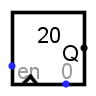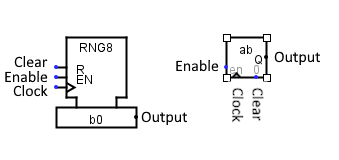#Random

 Library: MemoryIntroduced: 2.3.0 Appearance:Logisim-clasique Logisim-Evolution  Logisim-HolyCross

## Behavior

This component iterates through a pseudorandom sequence of numbers, which steps forward to the following number in the sequence each time the clock is triggered while the component is enabled. Technically speaking, the algorithm used to compute the pseudorandom sequence is a linear congruential generator: Starting from a seed r0, the following number r1 is the number

r1 = (25,214,903,917 r0 + 11) mod 248

The next value r2 is computed from r1 using the same computation, and so forth. This sequence is of 48-bit numbers; the value seen from the component is the low-order bits as configured by its Data Bits attribute, after first throwing out the lower 12 bits of the current seed.

Besides the clock input, the component also includes an enable input, which leads the clock input to be ignored when enable is 0, and the reset input, which resets the component's value asynchronously to the initial seed r0.

The initial seed is user-configurable. If it is configured at 0 (which is the default), then the seed is based on the current time; when instructed to reset through the reset input, the component computes a new seed based on the new current time.

## PinsThe Appearance attribute allows two different images for this component. Logisim Evolution presents inputs to the west and outputs to the east, while I present the pins from top to bottom and from west to east.

Input Clear: Labeled R
When this is 1, the pseudorandom sequence asynchronously resets to the initial seed. If seed is 0, this new seed should be different from the initial seed used previously the output will be initialized differently each time, as it depends on the time of day,. If set to 1, the output will be initialized to the first number in the series. Bit width 1.
Input Enable: labeled EM
The component is enabled when this input is 1 or indefined U; but if it is 0, then the clock input is ignored. Bit width 1.
Input Clock: labeled with a triangle
At the instant that this is triggered as specified by the Trigger attribute, the component steps to the following number in its sequence. Bit width 1.
Output:
Outputs the value currently stored by the component. Bit width matches Data Bits attribute.

## Attributes

When the component is selected or being added, Alt-0 through Alt-9 alter its Data Bits attribute.

Data Bits
The bit width of the value emitted by the component.
Seed
The starting value used for the pseudorandom sequence. If this is 0 (the default), then the starting value is based on the time that the random sequence began.
Trigger
Configures how the clock input is interpreted. The value :
rising edge indicates that the component should update its value at the instant when the clock rises from 0 to 1.
falling edge value indicates that it should update at the instant the clock falls from 1 to 0.
Label
The text within the label associated with the component.
Label Font
The font with which to render the label.

None.

## Text Tool Behavior

Allows the label associated with the component to be edited.

Back to Library Reference